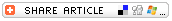## Search form# Guess-timating FunSubjects

• Mathematics
--Applied Math
--Arithmetic
--Statistics

• K-2
• 3-5
• 6-8
• 9-12

Brief Description

Fun activity reinforces concepts of estimation, number lines, mean, median, and mode.

Objectives

Students

• develop estimation skills.
• use data to build a number line.
• calculate the mean, median, and mode of a series of numbers.

Keywords

estimate, estimating, mean, median, mode, average, number line

• sheets of scrap paper (blank on one side)
• markers or crayons

Lesson Plan

This is a fun activity that reinforces many math skills. You could do this activity once a week to reinforce skills related to estimating, creating a number line, and/or calculating the mean, median, and mode of a series of numbers.

Introduce an "estimate-the-number of" jar. Any jar or glass container will work well. Fill the jar with peanuts (in the shell), M&Ms, crayons, gummy candies, small toys, crayons, or any other convenient item or product. You might give students a day or a week to guess-timate the number of items in the jar.

Provide each student with a sheet of paper. (This activity is a good one for making use of scrap paper -- leftover sheets of paper that are printed on only one side. If the paper is 8-1/2 x 11 inches in size, cut it in half.) Each student uses a crayon or marker to write his or her estimate on the paper. Encourage students to write their estimates on the paper in large and bold print.

When all students have written their estimates of the number of items in the jar, have them line up side-by-side around the room, in the hallway, or outdoors. Have them hold up their boldly written estimates so their classmates can see them. Have students arrange themselves into a "human number line" from the lowest estimate to the highest one. (You might challenge them to do without saying a word to each other.)

Next, at some grade levels, you might reinforce math skills by having students identify and record

• the range of estimates (the lowest and highest estimates; for example, students' estimates might range from 75 to 167).
• the median (the number in the middle). The same number of students should be standing to the left and right of the person holding the number that is the median. Another way to discern the median is to pair up the first and last student in the human number line. Then pair up the second and next to the last student, the third and third to last student the last student standing will represent the "median." If you have an even number of students, the median is the number that is halfway between the estimate of the two students at the center of the line; in other words, the median is the mean (average) of those two numbers at the center.
• the mode (the number that was guessed by the most students).
• the mean, or average (the total of all estimates divided by the number of students/estimates). It might be difficult for students to calculate the average of a large bank of data, so you might figure the estimate as a class; "do the math" together or project an online calculator onto a screen and use the calculator to figure the average.

Finally, reveal the actual number of items in the jar and determine

• which student's estimate was closest to the actual number. (You might ask the student to identify any "tips" he or she has that will help others as they create future estimates.)
• which number -- the median, mode, or mean -- came closest to matching the actual number of items.

This is a fun activity to repeat on a weekly basis to reinforce the concepts of range, mean, median, and mode. It also helps students develop their skills of estimation. Change the item in the jar each week.

Assessment

If you use this as a weekly activity, you might have students work on their own to identify the mean, median, mode, and range of the numbers on the week's number line of estimates. Have them write their responses on a small slip of paper; use that paper to give a quiz grade. You might give the student whose estimate is closest to the actual number a "free night of homework" pass or a certificate good for 10 points on the next quiz or test.

Lesson Plan Source

Education World

Submitted By

Gary Hopkins

National Standards

MATHEMATICS: Number and Operations
NM-NUM.PK-2.1 Understand Numbers, Ways of Representing Numbers, Relationships Among Numbers, and Number Systems
NM-NUM.PK-2.3 Compute Fluently and Make Reasonable Estimates
NM-NUM.3-5.1 Understand Numbers, Ways of Representing Numbers, Relationships Among Numbers, and Number Systems
NM-NUM.3-5.3 Compute Fluently and Make Reasonable Estimates
NM-NUM.6-8.1 Understand Numbers, Ways of Representing Numbers, Relationships Among Numbers, and Number Systems
NM-NUM.6-8.3 Compute Fluently and Make Reasonable Estimates
NM-NUM.9-12.1 Understand Numbers, Ways of Representing Numbers, Relationships Among Numbers, and Number Systems
NM-NUM.9-12.3 Compute Fluently and Make Reasonable Estimates

MATHEMATICS: Representation
NM-REP.PK-12.3 Use Representations to Model and Interpret Physical, Social, and Mathematical Phenomena

See more Lesson Plans of the Day in our Lesson Plan of the Day Archive. (There you can search for lessons by subject too.) For additional math lesson plans, see these Education World resources:

• Lesson Planning: Math
• Math Subject Center
• Teacher-Submitted Lesson Plans: Math
• Calculator Lessons from Texas Instruments
• Math Cross Puzzles
• It All Adds Up Puzzles
• Work Sheets from Teacher Created Materials: Math

Education World®
•